In this video, we are going to look at how to simplify numbers raised to negative exponents. After you finish this lesson, view all of our Algebra 1 lessons and practice problems.

## Example of Negative Exponents

To simplify the following: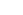$4^{-2}$
we have to move the 4 to the denominator and make the exponent positive.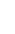$\dfrac{1}{4^2}$
Then, multiply the 4 by itself, 2 times which would simplify to: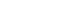$4 \times 4=16$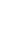$\dfrac{1}{16}$
To simplify the following: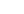$x^{-3}$
we have to move the x to the denominator and make the exponent positive.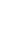$\dfrac{1}{x^3}$
If there is something more complex such as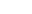$5x^{-2}$ then only the x will go to the denominator since it is the one with the negative exponent. This will be simplified to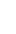$\dfrac{5}{x^2}$

Other examples: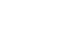$x^{-b}= \dfrac{1}{x^b}$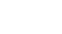$8^{-3}= \dfrac{1}{8^3}$

## Video-Lesson Transcript

Let’s have a quick look at negative exponents.

Here we have$4^{-2}$

If this is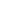$4^2$, it’s just going to be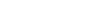$4^2 = 4 \times 4 = 16$

But here we have a negative exponent.

This has a different meaning.

We’re still going to do$4^2$ but the negative is going to change where we do that.

So whenever we have a number raised to a negative exponent, we’re going to move it to the denominator and make the exponent positive.

In this case, we’ll have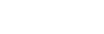$4^{-2} = \dfrac{1}{4^2} = \dfrac{1}{16}$

Notice that our answer is not negative. The negative in the exponent has nothing to do with our final answer.

It just shows where we’re going to do$4^2$. We’re going to move it to the denominator.

Let’s look at another one.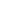$3^{-2}$

So, we’ll just move it to the denominator.$3^{-2} = \dfrac{1}{3^2}$

If you’re wondering where our numerator came from, it’s a regular thing. Since$3^{-2}$ is the same as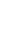$\dfrac{3^{-2}}{1}$.

So let’s continue solving.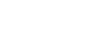$3^{-2} = \dfrac{1}{3^2} = \dfrac{1}{9}$

Let’s have another one.$5^{-3}$

So we’ll do the same thing. we’ll move it to the denominator and change the exponent to be positive.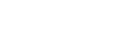$\dfrac{5^{-3}}{1} = \dfrac{1}{5^3} = \dfrac{1}{125}$

And the same is true for variables.

We have$x^{-3}$,

this is simply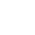$= \dfrac{1}{x^3}$The reason why I’m teaching it as numerator-denominator style is that it may become more complex.

If we have$5x^{-2}$

the thing here is that the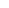$x$ is the only one raised to a negative exponent. Not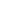$5$.

So when we solve this,$5$ stays on the numerator and the$x^{-2}$ will be on the denominator and change the exponent to positive.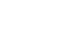$5x^{-2} = \dfrac{5}{x^2}$

Let’s have another one.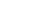$3x^{-2} y^3$

Here, we’ll have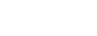$\dfrac{3x^{-2} y^3}{1} = \dfrac{3y^3}{x^2}$

The only variable to change to be a denominator is$x^{-2}$, the rest stays where they are.

Let’s have another example.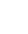$\dfrac{2}{x^-2}$

In all the other cases, we have a negative exponent but all are in the numerator.

In this case, we have a negative exponent on the denominator.

So what we’ll do is to do the opposite.

We’re going to place the negative exponent to the numerator then change the exponent to positive.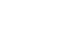$\dfrac{2}{x^-2} = 2x^2$

So wherever the term with a negative exponent is written, put it to the opposite location.

If it’s on the numerator, put it to the denominator and make the exponent positive.

If it’s on the denominator, put it to the numerator and make the exponent positive.

Keep in mind that the negative exponent does not affect the sign of the answer.